# 【win32】计算机图形学——中点法画线和八分法画圆

win32程序里面画线、画图和画矩形是极其简单，都有封装好的方法，一行Rectagle()、Ellipse()等等就完了，下面做这个画图程序，主要是说明如果利用计算机图形学的原生代码，中点法画线和八分法画圆，为了更有趣一点，还提供的颜色选择，以此说明一个普通的win32的制作过程。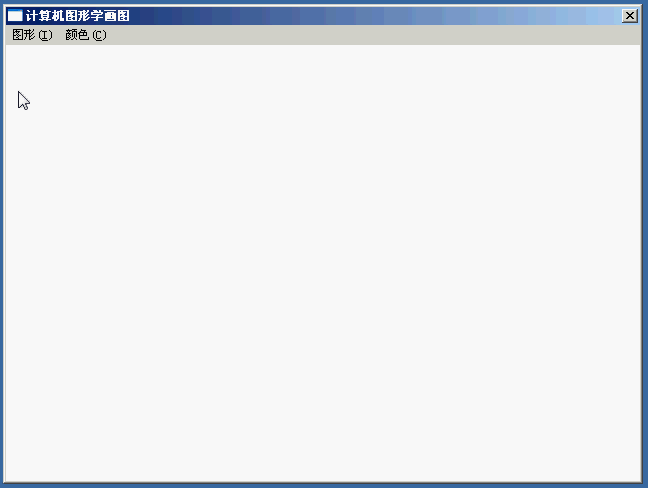1、如同《【win32】vs2010的窗体程序Helloworld》（点击打开链接）新建完一个win32程序之后，我们先要对程序进行一些小修改，诸如程序栏标题、菜单，删去最大最小化按钮以避免重绘的修改和删除对话框。

//自己定义的菜单项
#define IDM_LINE 201
#define IDM_CIRCLE 202
#define IDM_BLACK 301
#define IDM_RED 302
#define IDM_GREEN 303
#define IDM_BLUE 304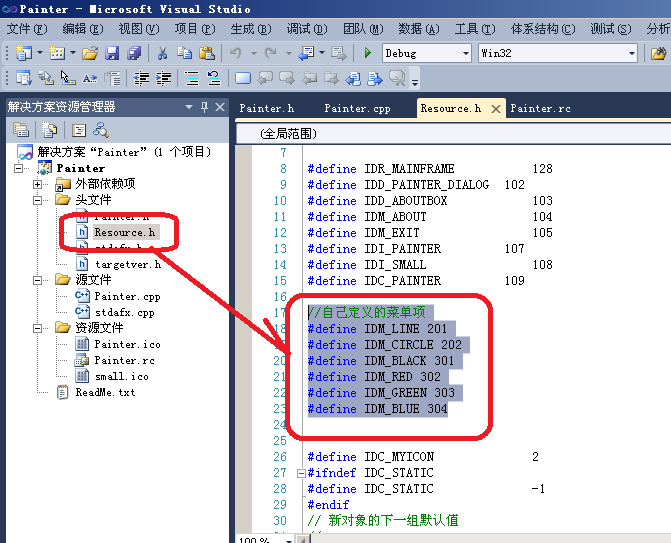//
// 菜单
//

BEGIN
POPUP "图形(&I)"
BEGIN
END
POPUP "颜色(&C)"
BEGIN
END
END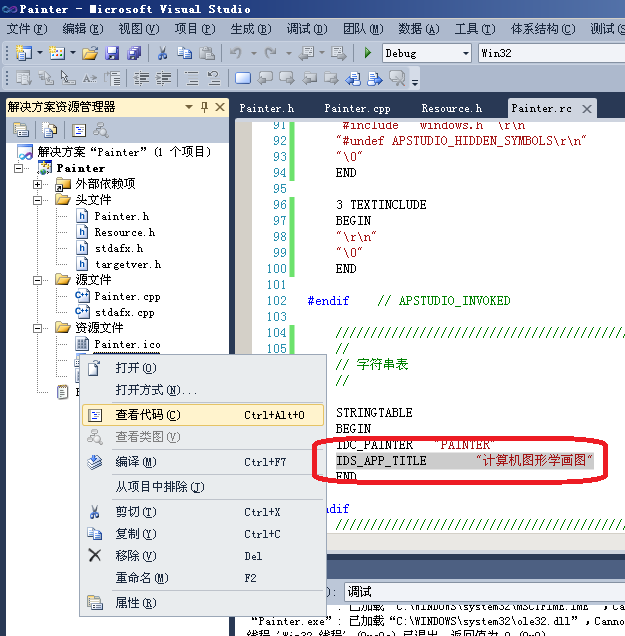2、之后就真的可以在Painter.h和Painter.cpp进行主要代码的编写了。

（1）首先是在Painter.h引入一个#include "math.h"，一会儿求两点距离开平方的时候要用到这个头文件，如下图所示：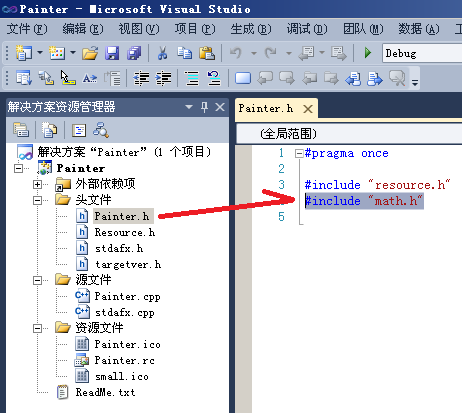（2）对于Painter.cpp中的BOOL InitInstance(HINSTANCE hInstance, int nCmdShow)程序初始化函数定义两个全局变量，用于记录用户选择的计算机图形学算法和颜色，并修改如下：

int algorithm;//记录用户当前选择的算法与使用的颜色
COLORREF color;//画笔颜色
BOOL InitInstance(HINSTANCE hInstance, int nCmdShow)
{
HWND hWnd;

hInst = hInstance; // 将实例句柄存储在全局变量中

hWnd = CreateWindow(szWindowClass, szTitle, WS_OVERLAPPEDWINDOW^WS_THICKFRAME^WS_MAXIMIZEBOX^WS_MINIMIZEBOX,
0, 0, 640, 480, NULL, NULL, hInstance, NULL);//调整窗口大小、样式，640*480的大小，在屏幕左上角打开，同时取出最大最小化按钮避免重绘

if (!hWnd)
{
return FALSE;
}

ShowWindow(hWnd, nCmdShow);
UpdateWindow(hWnd);

/*默认是画线和黑色*/
//令此菜单单选，第一个参数是菜单句柄、第二、三个参数是指明这个菜单从哪个编号到哪个编号是单选的，第四个参数是选择谁
algorithm=IDM_LINE;
color=RGB(0,0,0);

return TRUE;
}

（3）之后将消息回调函数LRESULT CALLBACK WndProc(HWND hWnd, UINT message, WPARAM wParam, LPARAM lParam)，先定义2个全局变量，用于记录鼠标的位置，并修改成如下：

POINT pointdown,pointup;//是一个结构体，储存鼠标x,y坐标
LRESULT CALLBACK WndProc(HWND hWnd, UINT message, WPARAM wParam, LPARAM lParam)
{
int wmId, wmEvent;
PAINTSTRUCT ps;
HDC hdc;

switch (message)
{
case WM_COMMAND:
wmId    = LOWORD(wParam);
wmEvent = HIWORD(wParam);
switch (wmId)//分析窗口的选择
{
case IDM_LINE:
//令此菜单单选，第一个参数是菜单句柄、第二、三个参数是指明这个菜单从哪个编号到哪个编号是单选的，第四个参数是选择谁
algorithm=IDM_LINE;
break;
case IDM_CIRCLE:
algorithm=IDM_CIRCLE;
break;
case IDM_BLACK:
color=RGB(0,0,0);
break;
case IDM_RED:
color=RGB(255,0,0);
break;
case IDM_GREEN:
color=RGB(0,255,0);
break;
case IDM_BLUE:
color=RGB(0,0,255);
break;
}
break;
case WM_PAINT:
hdc = BeginPaint(hWnd, &ps);
// TODO: 在此添加任意绘图代码...
EndPaint(hWnd, &ps);
break;
case WM_DESTROY:
PostQuitMessage(0);
break;

case WM_LBUTTONDOWN:
{
pointdown.x= LOWORD(lParam);//记录按下鼠标坐标信息
pointdown.y= HIWORD(lParam);
}
break;
case WM_LBUTTONUP:
{
pointup.x= LOWORD(lParam);//记录松开鼠标坐标信息
pointup.y= HIWORD(lParam);
HDC hdc=GetDC(hWnd);  //HDC是Windows的设备描述表句柄，此处指对话框的客户区
switch(algorithm){
case IDM_LINE:{//必须有这个括号，不然会提示变量不能在case中初始化的错误
int x1=pointdown.x, x2=pointup.x;
int y1=pointdown.y, y2=pointup.y;
float k=1.0*(y2-y1)/(x2-x1); //斜率
int flag=0;//是否沿y=x翻转
if (k>1||k<-1){//根据要斜率，判断直线是否超过y=x或者y=-x这条直线，是以x为底基还是以y为底基
flag=1;
x1^=y1^=x1^=y1;
x2^=y2^=x2^=y2;
k=1.0*(y2-y1)/(x2-x1);
}
float d=0.5-k;//初始值
if (x1>x2){
x1^=x2^=x1^=x2;
y1^=y2^=y1^=y2;
}
while(x1!=x2){//主位移，每次都像素+1
if(k>0&&d<0){//正向
++y1,++d;
}
else if(k<0&&d>0){//负向
--y1,--d;
}
d-=k;
++x1;
if(flag){
SetPixel(hdc,y1,x1,color);//翻转像素点
}
else{
SetPixel(hdc,x1,y1,color);
}
}
break;
}
case IDM_CIRCLE:{
int ox=(pointdown.x+pointup.x)/2,oy=(pointdown.y+pointup.y)/2;//根据用户两次按下鼠标的坐标，求圆的重点
float r=sqrt(((pointdown.x-pointup.x)*(pointdown.x-pointup.x)+(pointdown.y-pointup.y)*(pointdown.y-pointup.y))*1.0)/2;
//根据用户两次按下鼠标的坐标，两点距离公式/2求半径，其中最后的*1.0是强制将这个参数转成开平方根sqrt()函数只接受的double型
//以下是八分法画圆
float d=1.25-r;
int x=0,y=r,fx=r/1.4;
while (x!=fx){
if(d<0){
d+=2*x+3;
}
else{
d+=2*(x-y)+5;
--y;
}
SetPixel(hdc,ox+x,oy+y,color);
SetPixel(hdc,ox+x,oy-y,color);
SetPixel(hdc,ox-x,oy+y,color);
SetPixel(hdc,ox-x,oy-y,color);
SetPixel(hdc,ox+y,oy-x,color);
SetPixel(hdc,ox+y,oy+x,color);
SetPixel(hdc,ox-y,oy+x,color);
SetPixel(hdc,ox-y,oy-x,color);
++x;
}
break;
}

}
ReleaseDC(hWnd,hdc);//释放句柄
}
break;
default:
return DefWindowProc(hWnd, message, wParam, lParam);
}
return 0;
}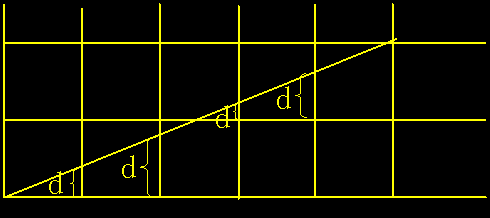X下标每增加1，d的值相应递增直线的斜率值k，即d＝d＋k。一旦d≥1，就把它减去1，这样保证d在0、1之间。当d≥0.5时，最接近于当前象素的右上方象素（Xi+1,Yi+1），而当d<0.5时，更接近于右方象素（Xi+1,Yi）。为方便计算，令e＝d-0.5，e的初值为-0.5，增量为k。当e≥0时，取当前象素（xi，yi）的右上方象素（Xi+1,Yi+1）。而当e<0时，更接近于右方象素（Xi+1,Yi+1）。可以改用整数以避免除法。由于算法中只用到误差项的符号，因此可作如下替换：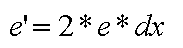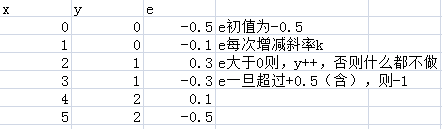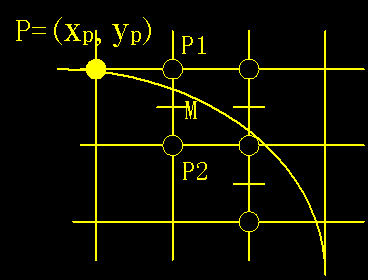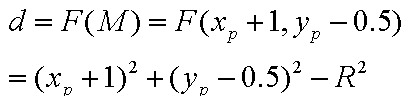©️2019 CSDN 皮肤主题: 编程工作室 设计师: CSDN官方博客